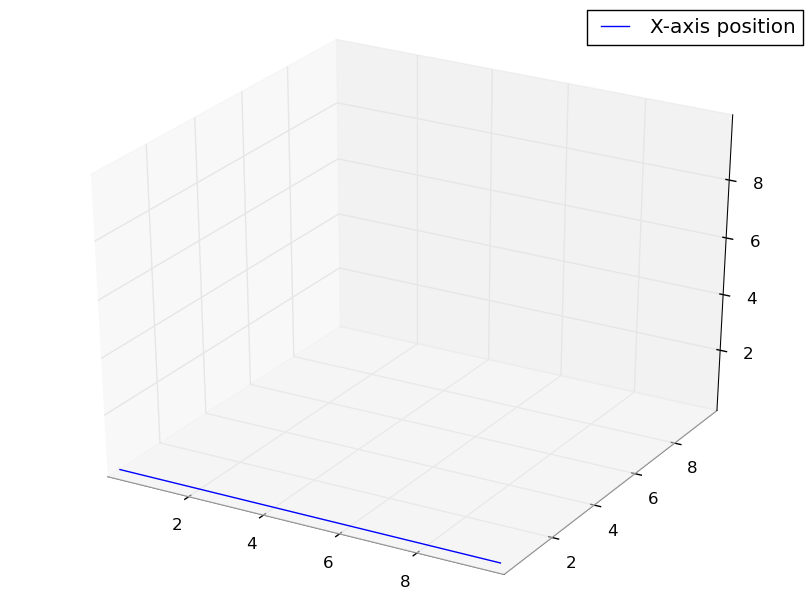# Bug in mplot3d set_xlim3d, set_ylim3d, set_zlim3d

Hello matplotlib-devs,

I found what I think is a bug.
If you run the following code, you’ll see a simple figure with a graph at the position where the x-axis should be.

Unfortunately this
is not the case, even if set_xlim3d(0,10) is set, so it should be at the edge of the plot.

The code:

import matplotlib as mpl

from mpl_toolkits.mplot3d import Axes3D

import
matplotlib.pyplot as plt

fig = plt.figure()

ax = Axes3D(fig)

x = range(11)

y = *11

z
= *11

ax.plot(x, y, z, label=‘X-axis position’)

ax.set_xlim3d(0,10)

ax.set_ylim3d(0,10)

ax.set_zlim3d(0,10)

ax.legend()

I attached the figure it produces.

You can also see, that from the edge to the 2 of one of the axis is a longer distance than
from 2 to 4 on the same axis.

A way to fix it is highly appreaciated.

Greetings
from Berlin

MartinYou should probably avoid
sending the same message to the list twice. You run the risk of only
annoying the developers and not getting any real help.

The mplot3d axes code is in a
major need of refactoring. There are very few developers who have worked
on the mplot3d code. But at the same time, you will find that the code is
much smaller, and more accessible than you might imagine. I found it very
easy to fix some bugs in the bar3d function that I needed for my project.

So I suggest you look into
fixing the bug yourself. It is pretty easy to submit patches to the
development team.

-Ben

[mailto:martin.bothe@…840…]

···

From: Martin Bothe
Sent: Thursday, April 15, 2010 11:11 AM
To: matplotlib-devel@lists.sourceforge.net
Subject: [matplotlib-devel] Bug in mplot3d set_xlim3d, set_ylim3d,
set_zlim3d

Hello matplotlib-devs,

I found what I think is a bug. If you run the following
code, you’ll see a simple figure with a graph at the position where the x-axis
should be.

Unfortunately this is not the case, even if set_xlim3d(0,10)
is set, so it should be at the edge of the plot.

The code:

import matplotlib as mpl

from mpl_toolkits.mplot3d import Axes3D

import matplotlib.pyplot as plt

fig = plt.figure()

ax = Axes3D(fig)

x = range(11)

y = *11

z = *11

ax.plot(x, y, z, label=‘X-axis position’)

ax.set_xlim3d(0,10)

ax.set_ylim3d(0,10)

ax.set_zlim3d(0,10)

ax.legend()

I attached the figure it produces.

You can also see, that from the edge to the 2 of one of the
axis is a longer distance than from 2 to 4 on the same axis.

A way to fix it is highly appreaciated.

Greetings from Berlin

Martin